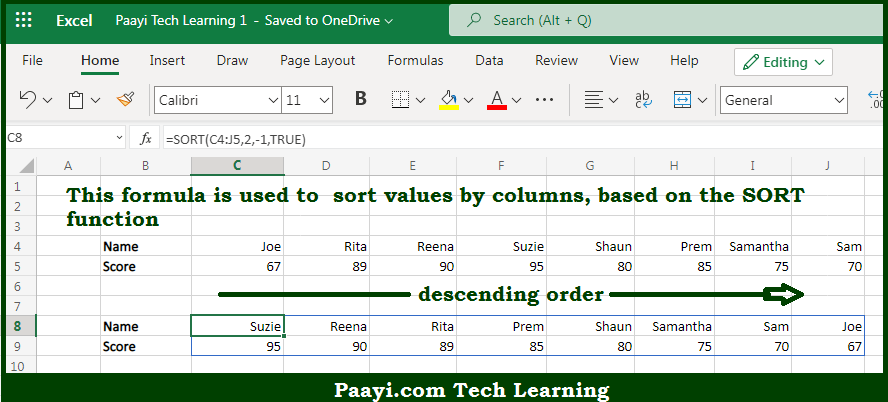# Learn How to Sort Values by Columns in Microsoft Excel

Written by | 0 Comments | 391 Views

In this article, you will learn how to evaluate things in Dynamic Arrays with formulas in Microsoft Excel using a single/combination(s) of functions. You will also know How to Sort Values by Columns and see the generic formula.

Learn How to Sort Values by Columns in Microsoft Excel

The main purpose of this formula is to sort values by columns. Here we will learn how to sort values by columns in the given data range in the workbook in Microsoft Excel. That implies, with the help of a formula based on the SORT function you can able to sort values by columns. So, with the help of this formula, you can able to sort values by columns in the given data range in the workbook in Microsoft Excel.

General Formula to Sort Values by Columns

=SORT(data,index,order,TRUE)

The Explanation to Sort Values by ColumnsSo we know that with the help of the given formula above you can able to sort values by columns. Here we will learn how to sort values by columns in the given data range in the workbook in Microsoft Excel. As we know that the SORTBY function allows the sorting based on one or more "sort by" arrays, as long as dimensions are compatible with the source data. So, with the help of this formula, you can able to sort values by columns. So now you have learned how to sort values by columns, in the given data range in the workbook in Microsoft Excel.